Electron. J. Differential Equations, Vol. 2017 (2017), No. 46, pp. 1-14.

### Pointwise bounds for positive supersolutions of nonlinear elliptic problems involving the p-Laplacian Asadollah Aghajani, Alireza Mosleh Tehrani

Abstract:
We derive a priori bounds for positive supersolutions of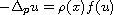, where p>1 and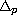is the p-Laplace operator, in a smooth bounded domain of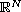with zero Dirichlet boundary conditions. We apply our results to the nonlinear elliptic eigenvalue problem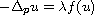, with Dirichlet boundary condition, whereis a nondecreasing continuous differentiable function on such that f(0)>0,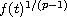is superlinear at infinity, and give sharp upper and lower bounds for the extremal parameter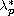. In particular, we consider the nonlinearities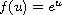and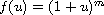(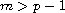) and give explicit estimates on. As a by-product of our results, we obtain a lower bound for the principal eigenvalue of the p-Laplacian that improves obtained results in the recent literature for some range of p and N.

Submitted June 10, 2015. Published February 14, 2017.
Math Subject Classifications: 35J66, 35J92, 35P15.
Key Words: Nonlinear eigenvalue problem; estimates of principal eigenvalue; extremal parameter.

Show me the PDF file (292 KB), TEX file for this article.Asadollah Aghajani School of Mathematics Iran University of Science and Technology Narmak, Tehran 16844-13114, Iran. phone +9821-73913426. Fax +9821-77240472 email: aghajani@iust.ac.ir Alireza Mosleh Tehrani School of Mathematics Iran University of Science and Technology Narmak, Tehran 16844-13114, Iran email: amtehrani@iust.ac.ir